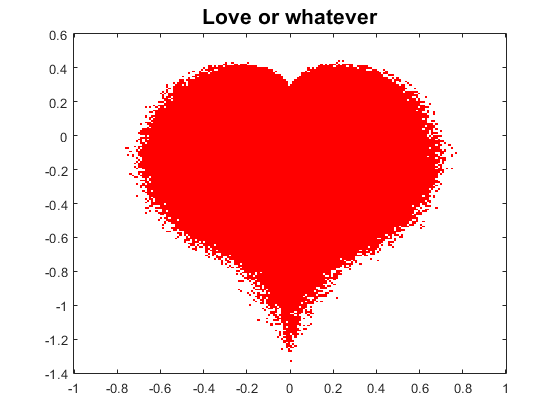# Happy Valentines Day!

I found this lovely algorithmic heart in the comments of an old Walking Randomly post. Research software superstar Mike Croucher gets credit for the blog, but the comment and the associated code are courtesy of Mariano Beguerisse (with some minor edits from me).
Thanks Mariano!
i = sqrt(-1);
% Fuzzy heart-shaped Mandelbrot fractal
% Mariano Beguerisse
% October 2010
% iterations
n = 300;
% resolution
N = 200;
% Create grid
x = linspace(-1, 1, N);
y = linspace(-1.4, .6, N);
[X,Y] = meshgrid(x, y);
Z = X + i*Y;
Zn = Z;
for j=1:n
% Mandelbrot map with random noise
Zn = -i*(Zn).^2 + (rand(N,N).^(1/5)).*Z;
M = abs(Zn);
ind1 = find(M<2);
ind2 = find(M>=2);
M(ind1) = 0;
M(ind2) = -1;
end
imagesc(x, y, M)
colormap([1 1 1; 1 0 0])
set(gca, YDir="normal")
title("Love or whatever", FontSize=16)|